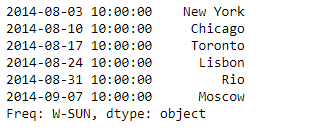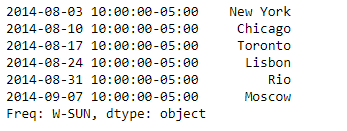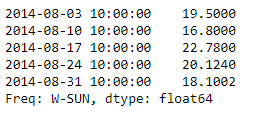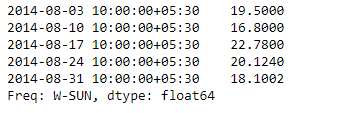# Python | Pandas Series.tz_localize

Python is a great language for doing data analysis, primarily because of the fantastic ecosystem of data-centric python packages. Pandas is one of those packages and makes importing and analyzing data much easier.

Pandas series is a One-dimensional ndarray with axis labels. The labels need not be unique but must be a hashable type. The object supports both integer- and label-based indexing and provides a host of methods for performing operations involving the index.

Pandas` Series.tz_localize()` function is used to localize tz-naive index of a Series or DataFrame to target time zone. This operation localizes the Index. In order to localize the values in a timezone-naive Series, we can use Series.dt.tz_localize().

Syntax: Series.tz_localize(tz, axis=0, level=None, copy=True, ambiguous=’raise’, nonexistent=’raise’)

Parameter :
tz : string or pytz.timezone object
axis : the axis to localize
level : If axis ia a MultiIndex, localize a specific level. Otherwise must be None
copy : Also make a copy of the underlying data
ambiguous : ‘infer’, bool-ndarray, ‘NaT’, default ‘raise’
nonexistent : str, default ‘raise’

Returns : Series or DataFrame

Example #1: Use `Series.tz_localize()` function to localize the time zone naive index of the given Series to the target time zone.

 `# importing pandas as pd ` `import` `pandas as pd ` ` `  `# Creating the Series ` `sr ``=` `pd.Series([``'New York'``, ``'Chicago'``, ``'Toronto'``, ``'Lisbon'``, ``'Rio'``, ``'Moscow'``]) ` ` `  `# Create the Datetime Index ` `didx ``=` `pd.DatetimeIndex(start ``=``'2014-08-01 10:00'``, freq ``=``'W'``,  ` `                                                 ``periods ``=` `6``)  ` ` `  `# set the index ` `sr.index ``=` `didx ` ` `  `# Print the series ` `print``(sr) `

Output :Now we will use `Series.tz_localize()` function to localize the given time zone naive index to time zone aware index. The target time zone is ‘US/Central’.

 `# Localize to 'US / Central' ` `sr.tz_localize(``'US/Central'``) `

Output :As we can see in the output, the `Series.tz_localize()` function has converted the given naive time zone index to a time aware index.

Example #2: Use `Series.tz_localize()` function to localize the time zone naive index of the given Series to the target time zone.

 `# importing pandas as pd ` `import` `pandas as pd ` ` `  `# Creating the Series ` `sr ``=` `pd.Series([``19.5``, ``16.8``, ``22.78``, ``20.124``, ``18.1002``]) ` ` `  `# Create the Datetime Index ` `didx ``=` `pd.DatetimeIndex(start ``=``'2014-08-01 10:00'``, freq ``=``'W'``,  ` `                                                 ``periods ``=` `5``)  ` ` `  `# set the index ` `sr.index ``=` `didx ` ` `  `# Print the series ` `print``(sr) `

Output :Now we will use `Series.tz_localize()` function to localize the given time zone naive index to time zone aware index. The target time zone is ‘Asia/Calcutta’.

 `# Localize to 'Asia/Calcutta' ` `sr.tz_localize(``'Asia/Calcutta'``) `

Output :My Personal Notes arrow_drop_upCheck out this Author's contributed articles.

If you like GeeksforGeeks and would like to contribute, you can also write an article using contribute.geeksforgeeks.org or mail your article to contribute@geeksforgeeks.org. See your article appearing on the GeeksforGeeks main page and help other Geeks.

Please Improve this article if you find anything incorrect by clicking on the "Improve Article" button below.

Article Tags :

Be the First to upvote.

Please write to us at contribute@geeksforgeeks.org to report any issue with the above content.